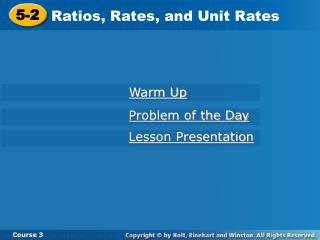DownloadDownload Presentation5-2

5-2

Download Presentation5-2

- - - - - - - - - - - - - - - - - - - - - - - - - - - E N D - - - - - - - - - - - - - - - - - - - - - - - - - - -
Presentation Transcript

1. 5-2 Ratios, Rates, and Unit Rates Course 3 Warm Up Problem of the Day Lesson Presentation

2. Learn to work with rates and ratios.

3. Vocabulary rate unit rate unit price

4. A rate is a comparison of two quantities that have different units. 90 3 Ratio: Read as “90 miles per 3 hours.” 90 miles 3 hours Rate:

5. 90 3 The ratio can be simplified by dividing: Unit rates are rates in which the second quantity is 1. 90 3 30 1 = 30 miles, 1 hour unit rate: or 30 mi/h

6. 30 words minute 1 2 30 words • 2 minute • 2 1 2 Additional Example 1: Finding Unit Rates Geoff can type 30 words in half a minute. How many words can he type in 1 minute? Write a rate. Multiply to find words per minute. 60 words 1 minute = Geoff can type 60 words in one minute.

7. Check It Out: Example 1 Penelope can type 90 words in 2 minutes. How many words can she type in 1 minute? 90 words 2 minutes Write a rate. Divide to find words per minute. 90 words ÷ 2 2 minutes ÷ 2 45 words 1 minute = Penelope can type 45 words in one minute.

8. YOUR TURN: Chemistry Application Five cubic meters of copper has a mass of 44,800 kilograms. What is the density of copper? 44,800 kg 5 m3 Write a rate. Copper has a density of_________________.

9. Check It Out: Example 2B A piece of gem stone with a volume of 0.25 cubic meters weighs 3540 kilograms. What is the density of the gem stone? 3540 kg 0.25 m3 Write a rate. 3540 kg • 4 0.25 m3 • 4 Multiply to find kilograms per 1 m3. 14,160 kg 1 m3 The gem stone has a density of 14,160 kg/m3.

10. 455 students 91 computers 468 students 91 computers  5 students 1 computer  Additional Example 3A: Estimating Unit Rates Estimate each unit rate. 468 students to 91 computers Choose a number close to 468 that is divisible by 91. Divide to find students per computer. 468 students to 91 computers is approximately 5 students per computer.

11. 312 feet 8 seconds 313 feet 8 seconds  39 feet 1 second  Additional Example 3B: Estimating Unit Rates Estimate each unit rate. 313 feet in 8 seconds Choose a number close to 313 that is divisible by 8. Divide to find feet per second. 313 feet to 8 seconds is approximately 39 feet per second.

12. 595 players 85 soccer balls 583 players 85 soccer balls  YOUR TURN! Estimate each unit rate. 583 soccer players to 85 soccer balls. Choose a number close to 583 that is divisible by 85. Divide to find players per soccer ball. 583 soccer players to 85 soccer balls is approximately ____ players per soccer ball.

13. 276 yards 3 hours 271 yards 3 hours  YOUR TURN! Estimate each unit rate. 271 yards in 3 hours Choose a number close to 271 that is divisible by 3. Divide to find yards per hour. 271 yards to 3 hours is approximately _____ yards per hour.

14. Unit price is a unit rate used to compare price per item.

15. Additional Example 4A: Finding Unit Prices to Compare Costs Pens can be purchased in a 5-pack for \$1.95 or a 15-pack for \$6.20. Which is the better buy? Divide the price by the number of pens. price for package number of pens \$1.95 5 =  \$0.39 price for package number of pens \$6.20 15 =  \$0.41 The better buy is the 5-pack for \$1.95.

16. price for jar number of ounces price for jar number of ounces YOUR TURN! Jamie can buy a 15-oz jar of peanut butter for \$2.19 or a 20-oz jar for \$2.78. Which is the better buy? Divide the price by the number of ounces. \$2.19 15 =  \$2.78 20 =  The better buy is the _____________________.

17. YOUR TURN! Golf balls can be purchased in a 3-pack for \$4.95 or a 12-pack for \$18.95. Which is the better buy? Divide the price by the number of balls. price for package number of balls \$4.95 3 =  price for package number of balls \$18.95 12  = The better buy is the ______________________.

18. price for bottle number of ounces price for bottles number of ounces YOUR TURN, AGAIN! John can buy a 24 oz bottle of ketchup for \$2.19 or a 36 oz bottle for \$3.79. Which is the better buy? Divide the price by the number of ounces. \$2.19 24 =  \$0.09 \$3.79 36 =  \$0.11 The better buy is the 24-oz jar for \$2.19.

19. Lesson Quiz Part 1 1. A penny has a mass of 2.5 g and a volume of approximately 0.360 cm3. What is the approximate density of a penny? 2. Meka can make 6 bracelets per half hour. How many bracelets can she make in 1 hour? Estimate the unit rate. 3. \$2.22 for 6 stamps 4. 8 heartbeats in 6 seconds

20. Lesson Quiz: Part 2 Determine the better buy. 5. A half dozen carnations for \$4.75 or a dozen for \$9.24 6. 4 pens for \$5.16 or a ten-pack for \$12.90.